• 多元正态分布 多元正态分布 多元正态分布 多元正态分布
• 多元正态分布一些性质的证明，比较详细。有兴趣的可以下去看看 多元正态分布一些性质的证明，比较详细。有兴趣的可以下去看看 多元正态分布一些性质的证明，比较详细。有兴趣的可以下去看看
• 多元正态分布原理及特点，很详细 从一维开始，然后二维，最后扩展到三维
• ## 透彻理解多元正态分布

千次阅读 多人点赞 2020-03-14 14:50:52
本篇内容主要是对于基本书籍教材多元正态分布相关章节所写的学习笔记，结合自己的理解尽可能表述得通俗易懂，主要思路内容取自《程序员的数学之概率统计》。 前言 多元正态分布就是含有多个变量的正态分布，为什么...
文章目录前言多元标准正态分布多元一般正态分布缩放与位移相同尺度缩放与位移不同尺度旋转变换多元正态分布的概率密度函数多元正态分布的性质
本篇内容主要是对于基本书籍教材多元正态分布相关章节所写的学习笔记，结合自己的理解尽可能表述得通俗易懂，主要思路内容取自《程序员的数学之概率统计》。
前言
多元正态分布就是含有多个变量的正态分布，为什么关于多元正态分布要专门写一篇学习笔记？因为其具有重要意义，在理论研究或者实际应用中，我们常会首先考虑多元正态分布是否适用，如果不符，再考虑其他类型的分布。基于下面两个特征，多元正态分布应用十分广泛：

多元正态分布的表达式易于处理，且理论推导的结果较为简洁
现实生活中很多问题都能通过多元正态分布解释或近似

多元正态分布的数学形式复杂，但大多情况下都可以通过椭圆或椭圆体表述，这就是学习时需要具备的几何理解。
多元标准正态分布
定义：如果列向量$Z=(Z_1,\dots,Z_n)^T$由n个遵从标准正态分布的随机变量$Z_1,\dots,Z_n$组成，那么称Z遵从n元标准正态分布。二元标准正态分布（均值0方差1）的概率密度及概率密度函数如下图所示：当$Z_1,\dots,Z_n$互相独立时，概率密度函数如下：
$f_{Z}(z)=g\left(z_{1}\right) g\left(z_{2}\right) \cdots g\left(z_{n}\right)$
这里的g是标准正态分布的概率密度函数，具体形式如下：
$f_{Z}(z)=c \exp \left(-\frac{z_{1}^{2}}{2}\right) \cdot c \exp \left(-\frac{z_{2}^{2}}{2}\right) \cdots c \exp \left(-\frac{z_{n}^{2}}{2}\right)$
这里的c是根据总概率为1这一条件所求得的常量。整理上式可得到如下表达式：
$f_{Z}(z)=d \exp \left(-\frac{1}{2}\|z\|^{2}\right)$
这就是n元标准正态分布的概率密度函数。d仍是由总概率为1的条件求得的常量。（$c\int_{-\infty}^{\infty} \exp \left(-z^{2} / 2\right) d z=1$，$c=1/\sqrt{2\pi}$；$d=c^n$这就是c和d的具体值）
观察n元标准正态分布的概率密度函数，向量z的长度为：
$\|z\|=\sqrt{z_{1}^{2}+z_{2}^{2}+\cdots+z_{n}^{2}}=\sqrt{z^{T} z}$
由此可发现，概率密度函数$f_Z(z)$的等高线是一个圆或者等值面试一个球面或超球面（这里超球面或者等值线就是所有函数值相同的点连接得到的图形）。
为什么是圆或者球面超球面可以这么理解：$f_Z(z)$的表达式中的自变量其实就是$||z||$，也就是说，即使不知道向量z具体值，只要确定其长度，就可以计算得到$f_Z(z)$。所以只要向量长度相同，函数值就相同，也就是以原点为中心的圆周上任意位置的函数值都相同，这就是球面超球面圆的定义。
确定Z的概率密度函数之后，再来求期望值向量与协方差矩阵。以n=3的情况为例：
$\begin{array}{l} \mathrm{E}[\boldsymbol{Z}]=\left(\begin{array}{c} \mathrm{E}\left[Z_{1}\right] \\ \mathrm{E}\left[Z_{2}\right] \\ \mathrm{E}\left[Z_{3}\right] \end{array}\right)=\left(\begin{array}{l} 0 \\ 0 \\ 0 \end{array}\right)=o \\ \mathrm{V}[\boldsymbol{Z}]=\left(\begin{array}{ccc} \mathrm{V}\left[Z_{1}\right] & \operatorname{Cov}\left[Z_{1}, Z_{2}\right] & \operatorname{Cov}\left[Z_{1}, Z_{3}\right] \\ \operatorname{Cov}\left[Z_{2}, Z_{1}\right] & \mathrm{V}\left[Z_{2}\right] & \operatorname{Cov}\left[Z_{2}, Z_{3}\right] \\ \operatorname{Cov}\left[Z_{3}, Z_{1}\right] & \operatorname{Cov}\left[Z_{3}, Z_{2}\right] & \mathrm{V}\left[Z_{3}\right] \end{array}\right)=\left(\begin{array}{lll} 1 & 0 & 0 \\ 0 & 1 & 0 \\ 0 & 0 & 1 \end{array}\right) \end{array}$
推广到n元的情况也是一样，期望值是n元零向量$o$，协方差矩阵是n元单位矩阵$I$。综上，即可通过$Z \sim N(o,I)$表示Z遵从n元标准正态分布。有以下性质：

各元素标准差均为1
不仅坐标轴方向，任意方向标准差都为1

这里由上面已证明的等高线是圆，可从第一条推出第二条。
多元一般正态分布
一般正态分布可由标准正态分布通过平移或缩放得到，同理，多元一般正态分布也可以由多元标准正态分布通过变换得到。在讨论之前先看一下如何通过转换随机变量X来获得需要的期望和方差。假如有两个随机变量Y和Z，他们与X的关系满足$Y=X+c$，$Z=cX$。那么他们的方差和均值变化的结果如下，先看Y的情况：
$E[Y]=E[X+c]=E[X]+c, V[Y]=V[X+c]=V[X]$
再看Z的情况：
$E[Z]=E[cX]=cE[X], V[Z]=V[cX]=c^2V[X]$
根据这些性质，就可以通过转换随机变量X来获得需要的期望值与方差了。例如X的期望为$\mu$方差为$\sigma^2$，此时只要令$W=\frac{X-\mu}{\sigma}$即可得到期望为0，方差为1的分布了。这个令期望为0方差为1的转换处理过程就叫做标准化（或者归一化）。
缩放与位移相同尺度
设$X=\sigma Z+\mu$，其中$\sigma$是一个正的常量，$\mu$是一个n元的常向量。此时，X的期望值与方差如下：
\begin{aligned} &\mathrm{E}[\boldsymbol{X}]=\sigma \mathrm{E}[\boldsymbol{Z}]+\boldsymbol{\mu}=\boldsymbol{\mu}\\ &\mathrm{V}[\boldsymbol{X}]=\sigma^{2} \mathrm{V}[\boldsymbol{Z}]=\sigma^{2} I=\left(\begin{array}{ccc} \sigma^{2} & & \\ & \ddots & \\ & & \sigma^{2} \end{array}\right) \end{aligned}
X服从的分布就称为“期望值为$\mu$且协方差矩阵为$\sigma^2 I$的n元正态分布”，记作$X \sim N(\mu, \sigma^2 I)$。二元情况下其概率密度与概率密度函数如下图所示：上面右图的体积为1，基准圆圆心变为$\mu$，半径变为$\sigma$。
缩放与位移不同尺度
上一部分的缩放中，所有方向的缩放程度是相等的。如果不同坐标轴缩放倍率不同，那就会得到一个椭圆状分布。基准圆也会变为椭圆。还是以列向量$Z=(Z_1,\dots,Z_n)^T$为例，如果对Z的各个元素分别缩放不同倍，得到$\boldsymbol{X} =\left(\sigma_{1} Z_{1}, \cdots, \sigma_{n} Z_{n}\right)^{T}$，这一变换的矩阵形式如下：
$\boldsymbol{X}=D \boldsymbol{Z}, \quad D \equiv\left(\begin{array}{ccc} \sigma_{1} & & \\ & \ddots & \\ & & \sigma_{n} \end{array}\right)$
此时X的协方差矩阵是如下对角阵：
$\mathrm{V}[\boldsymbol{X}]=D^{2}=\left(\begin{array}{ccc} \sigma_{1}^{2} & & \\ & \ddots & \\ & & \sigma_{n}^{2} \end{array}\right)$
协方差矩阵的求解推导过程如下（如果对下面推导过程不明白，可参考之前博文协方差与协方差矩阵内容）：
$\mathrm{V}[\boldsymbol{X}]=\mathrm{V}[D \boldsymbol{Z}]=D \mathrm{V}[\boldsymbol{Z}] D^{T}=D I D^{T}=D^{2}$
如果再加上常向量$\mu$来使其在各个坐标轴方向上平移不同的距离，那么期望值向量会增加$\mu$，而协方差矩阵不变。此时的分布就是一般的多元正态分布的形式，记作$N(\mu,D^2)$。其概率密度与概率密度函数如下图所示：旋转变换
旋转已有的分布后得到的将是更加一般的多元正态分布，通常我们使用正交矩阵的乘法运算来表示旋转变换。如果X是以原点为中心的多元正态分布，有正交矩阵Q使得$Y=QX$，那么：
$\begin{array}{l} \mathrm{E}[Y]=Q \mathrm{E}[X]=o \\ \mathrm{V}[Y]=Q \mathrm{V}[X] Q^{T}=Q D^{2} Q^{T} \end{array}$
由此就得到了一个协方差矩阵不是对角阵的一般多元正态分布。反之，如果希望某个分布属于多元正态分布（如果希望得到的多元正态分布具有符合要求的协方差矩阵V），那么就令其协方差矩阵符合$V=QD^2Q^T$这个条件，其中Q为正交阵，D为对角阵。
注意，这个条件和$Q^TVQ=D^2$是等价的。又因为协方差矩阵V是一个对称阵，所以这个条件可以描述为：对于给定的对称阵V，找到一个合适的正交矩阵Q，使得$Q^TVQ$是一个对角阵。这就是通过对称矩阵和正交矩阵实现矩阵对角化的方法。依据的是该定理：如果一个矩阵H是对称矩阵，那么必然存在正交矩阵Q，使得$Q^THQ$为对角阵。这个对角阵的每一个对角元素都是特征值，每个特征值对应的Q中的向量都是特征向量。
解出对角阵后只需使$D^{2}=\operatorname{diag}\left(\lambda_{1}, \cdots, \lambda_{n}\right)$即可解得D为：
$D = \left(\begin{array}{ccc} \sqrt{\lambda_{1}} & & \\ & \ddots & \\ & & \sqrt{\lambda_{n}} \end{array}\right)$
由此将D与Q代入上面的V的表达式中，即可得到多元正态分布$N(o, V)$。再加上常向量$\mu$即可实现平移，得到最一般的多元正态分布$N(\mu,V)$。其概率密度与概率密度函数示意图如下：多元正态分布的概率密度函数
为讨论多元正态分布的各种方便的性质，首先需要知道多元正态分布的概率密度函数。对于n元标准正态分布上面已经推导过其概率密度函数如下：
$f_{Z}(z)=\frac{1}{\sqrt{2 \pi}^{n}} \exp \left(-\frac{1}{2}\|z\|^{2}\right)$
如何对Z进行变换，从而得到一个协方差矩阵为$V=QD^2Q^T$的一般n元正态分布呢？
先令
$Y=QDZ$
这里的Q是正交阵，D是对角线元素全部都为正的对角阵。由于Q和D都是正规矩阵，所以它们的乘积QD也是正规矩阵。
这里再补充一个知识点，即多变量乘以一个正规矩阵变换之后的新变量的概率密度函数和原概率密度函数的关系。该知识点可进行如下描述。
对于$Z_1=g_1(X_1,\dots,X_n), \dots, Z_n=g_n(X_1,\dots,X_n)$的概率密度函数f有以下结论：
$f_{Z_{1}, \cdots, Z_{n}}\left(z_{1}, \cdots, z_{n}\right)=\left|\frac{\partial\left(x_{1}, \cdots, x_{n}\right)}{\partial\left(z_{1}, \cdots, z_{n}\right)}\right| f_{X_{1}, \cdots, X_{n}}\left(x_{1}, \cdots, x_{n}\right)$
其中
$\frac{\partial\left(x_{1}, \cdots, x_{n}\right)}{\partial\left(z_{1}, \cdots, z_{n}\right)} \equiv \operatorname{det}\left(\begin{array}{ccc} \frac{\partial x_{1}}{\partial z_{1}} & \cdots & \frac{\partial x_{1}}{\partial z_{n}} \\ \vdots & & \vdots \\ \frac{\partial x_{n}}{\partial z_{1}} & \cdots & \frac{\partial x_{n}}{\partial z_{n}} \end{array}\right)=\frac{1}{\partial\left(z_{1}, \cdots, z_{n}\right) / \partial\left(x_{1}, \cdots, x_{n}\right)}$
这个知识点讲述了这么一个情况：以二维为例，x和y组成了表示概率密度的平面网格点，而z轴则是概率密度函数值，其和xy平面围成的体积必须为1。当把xy进行线性变换之后，网格的大小也会有倍数的扩大，这个面积的变化就叫做面积扩大率，其数值为$|detA|$。既然面积扩大了，那么为了维持体积不变，概率密度函数值就需要缩小相应的倍数。所以，多元标准正态分布的随机变量乘以$A=QD$之后，概率密度函数就会改变为：
$f_{\boldsymbol{Y}}(\boldsymbol{y})=\frac{1}{|\operatorname{det} A|} f_{\boldsymbol{Z}}\left(A^{-1} \boldsymbol{y}\right)=\frac{1}{|\operatorname{det} A|} \cdot \frac{1}{\sqrt{2 \pi}^{n}} \exp \left(-\frac{1}{2}\left\|A^{-1} \boldsymbol{y}\right\|^{2}\right)$
这还不够，这个概率密度函数中体现不出方差的概念。对上面等式右边进行整理，尝试用协方差矩阵V来表示。首先根据下面关系（如果对下面推导过程不明白，可参考之前博文协方差与协方差矩阵内容）：
$V=\mathrm{V}[A Z]=A \mathrm{V}[Z] A^{T}=A I A^{T}=A A^{T}$
可得到如下结论
$\operatorname{det} V=\operatorname{det}\left(A A^{T}\right)=(\operatorname{det} A)\left(\operatorname{det} A^{T}\right)=(\operatorname{det} A)^{2}$
又由于$V^{-1}=\left(A A^{T}\right)^{-1}=\left(A^{T}\right)^{-1} A^{-1}=\left(A^{-1}\right)^{T} A^{-1}$，可得到如下结果
$\left\|A^{-1} \boldsymbol{y}\right\|^{2}=\left(A^{-1} \boldsymbol{y}\right)^{T}\left(A^{-1} \boldsymbol{y}\right)=\boldsymbol{y}^{T}\left(A^{-1}\right)^{T} A^{-1} \boldsymbol{y}=\boldsymbol{y}^{T} V^{-1} \boldsymbol{y}$
综上，最终结果为：
$f_{Y}(y)=\frac{1}{\sqrt{(2 \pi)^{n} \operatorname{det} V}} \exp \left(-\frac{1}{2} y^{T} V^{-1} y\right)$
这就得到了期望值为o的n元正态分布$N(o,V)$的概率密度函数。
这里还有局限性，因为期望值是0，为得到更一般的多元正态分布概率密度函数表达式，令Y位移至$\hat Y=Y+\mu$，即可得到期望值为$\mu$的n元正态分布了，由于只是位移，所以面积和体积都不会发生变化，于是其概率密度函数变化如下
$f_{\tilde{Y}}(\tilde{y})=f_{Y}(\tilde{y}-\mu)=\frac{1}{\sqrt{(2 \pi)^{n} \operatorname{det} V}} \exp \left(-\frac{1}{2}(\tilde{y}-\mu)^{T} V^{-1}(\tilde{y}-\mu)\right)$
综上，最终可得到n元正态分布的概率密度函数为：
$f(\boldsymbol{x})=\frac{1}{\sqrt{(2 \pi)^{n} \operatorname{det} V}} \exp \left(-\frac{1}{2}(\boldsymbol{x}-\boldsymbol{\mu})^{T} V^{-1}(\boldsymbol{x}-\boldsymbol{\mu})\right)$
如果觉得上面公式太复杂，可以抽象为如下形式：
$f(x)=C \exp(X元素的二次式)$
反之，如果概率密度形如上式，则就可以确定X的分布是一种正态分布。这与一元情况同理，对二次式进行配平得方差期望，之后根据体积为1求得常量。
多元正态分布的性质
多元正态分布具有下面三个良好的性质。

可由期望值向量和协方差矩阵确定具体分布
如果各随机变量不相关，则一定独立
多元正态分布经过线性变换之后还是多元正态分布
多元正态分布的条件分布也是多元正态分布
多元正态分布的边缘分布也是多元正态分布

对于第一条性质，显然成立。只要计算期望值向量和协方差矩阵将其代入上一部分求出的概率密度函数公式即可解得概率密度函数。
对于第二条性质，解释之前首先要明确一个概念：
如果随机变量X和Y相互独立，那么其协方差为0，即相关系数为0。
如果随机变量X和Y的协方差为0，即相关系数为0，那么X和Y不一定相互独立。
但是，如果X和Y组成二元正态分布，就可以由协方差等于0直接推得X与Y独立。理由如下。
因为协方差是0，所以协方差矩阵V为对角阵，所以其逆矩阵也是对角阵，两个随机变量方差分别为$\sigma$和$\tau$，那么
\begin{aligned} f_{X}(x) &=\operatorname{\square exp}\left(-\square(x-\mu)^{T} V^{-1}(x-\mu)\right)=\square \exp \left(-\square \frac{(x-\mu)^{2}}{\sigma^{2}}-\square \frac{(y-\nu)^{2}}{\tau^{2}}\right) \\ &=\operatorname{\square exp}\left(-\square \frac{(x-\mu)^{2}}{\sigma^{2}}\right) \exp \left(-\square \frac{(y-\nu)^{2}}{\tau^{2}}\right) \end{aligned}
其中$\square$代表无关紧要的常量，上式可以分解为仅含x的式子和仅含y的式子。这就表明了两个随机变量相互独立，为什么呢？

这里看一下独立性的定义。
独立性有多种表述方式，其中最易于理解的就是“无论是否附加条件，分布都不会发生变化”，那对于随机变量X和Y，这个表述的数学表达形式就是，如果下式始终成立，则称X与Y独立。
$f_{Y|X}(b|a)=f_Y(b)$
这个表达式等价于：
$f_{X,Y}(a,b)=f_X(a)f_Y(b)$

如果随机变量超过两个，该结论依然成立。因为最终$V[X]$是一个对角阵，只要它是对角阵，就可以将概率密度函数分解为仅含单个随机变量的n个正态分布概率密度函数的乘积。
对于第三条性质，对于$\boldsymbol{X} \sim \mathrm{N}(\boldsymbol{\mu}, V)$，假设（正规矩阵）A是一个取值确定的矩阵，经过变量变换$Y=AX$将得到一个n元正态分布，变换后的期望值和协方差矩阵如下（如果对下面推导过程不明白，可参考之前博文协方差与协方差矩阵内容）：
\begin{aligned} \boldsymbol{\nu} & \equiv \mathrm{E}[\boldsymbol{Y}]=A \mathrm{E}[\boldsymbol{X}]=A \boldsymbol{\mu} \\ W & \equiv \mathrm{V}[\boldsymbol{Y}]=A \mathrm{V}[\boldsymbol{X}] A^{T}=A V A^{T} \end{aligned}
由于Y具有如下概率密度函数，所以可确认它是一个多元正态分布。
\begin{aligned} f_{Y}(\boldsymbol{y}) &=\frac{1}{|\operatorname{det} A|} f_{X}\left(A^{-1} y\right) \\ &=\square \exp \left(-\frac{1}{2}\left(A^{-1} \boldsymbol{y}-\boldsymbol{\mu}\right)^{T} V^{-1}\left(A^{-1} \boldsymbol{y}-\boldsymbol{\mu}\right)\right)\\ &=\square \exp \left(y的元素的二次式\right) \end{aligned}
对于第四条性质，可以用截面的形式来解读，性质重新描述如下。
假设$\boldsymbol{X} \equiv\left(X_{1}, X_{2}, \cdots, X_{n}\right)^{T}$遵从n元正态分布$N(o,V)$。在$X_1=c$的条件下，由剩余向量组成的c的条件分布将是一个n-1元正态分布。
接下来是验证，设V的逆矩阵中的元素为r，条件概率密度函数可写为：
$\begin{array}{l} f_{\tilde{X} | X_{1}}\left(x_{2}, \cdots, x_{n} | c\right) \\ =\square \exp \left(-\frac{1}{2}\left(c, x_{2}, \cdots, x_{n}\right)\left(\begin{array}{cccc} r_{11} & r_{12} & \cdots & r_{1 n} \\ r_{21} & r_{22} & \cdots & r_{2 n} \\ \vdots & \vdots & & \vdots \\ r_{n 1} & r_{n 2} & \cdots & r_{n n} \end{array}\right)\left(\begin{array}{c} c \\ x_{2} \\ \vdots \\ x_{n} \end{array}\right)\right) \\ =\square \exp (x_{2}, \cdots, x_{n}的二次式) \end{array}$
通过类似证明，反复应用该结论，就可证明所有由剩余向量组成的条件分布都是多元正态分布。引入n=3的例子来看看直观理解，当n=3时，三元正态分布的概率密度函数的等值面是椭圆体，截面上就是椭圆，也就是二元正态分布，示意图如下：如果要计算条件分布的期望值向量与协方差矩阵的值，应用如下通用结论即可。对于这样的分布：
$\left(\begin{array}{l} X \\ Y \end{array}\right) \sim \mathrm{N}\left(\left(\begin{array}{l} \mu \\ \mu \end{array}\right),\left(\begin{array}{ll} 甲 & 乙 \\ 乙^{T} & 丁 \end{array}\right)\right)$
如果给定X=c，Y的条件分布为$\mathrm{N}(\tilde{\boldsymbol{\nu}}, \tilde{W})$，其中：
\begin{aligned} \tilde{\nu} & \equiv \nu+乙^{T} 甲^{-1}(c-\mu) \\ \tilde{W} & \equiv 丁-乙^{T} 甲^{-1} 乙 \end{aligned}
这里的甲乙丁都是矩阵。

对于第五条性质，可以通过积分计算边缘分布的概率密度函数，通过观察积分可发现，边缘分布其实也是一个多元正态分布。其期望值和协方差矩阵的值很容易就能得到，例如设$\boldsymbol{X}=\left(X_{1}, X_{2}, X_{3}, X_{4}\right)^{T}$，且$\boldsymbol{\tilde X}=\left(X_{2}, X_{3}, X_{4}\right)^{T}$，相应的期望值向量与协方差矩阵如下。
$\begin{array}{l} \mathrm{E}[\boldsymbol{X}]=\left(\begin{array}{c} \mathrm{E}\left[X_{1}\right] \\ \hline \mathrm{E}\left[X_{2}\right] \\ \mathrm{E}\left[X_{3}\right] \\ \mathrm{E}\left[X_{4}\right] \end{array}\right)=\left(\begin{array}{c} *\\ \hline \\ \mathrm{E}[\tilde{\boldsymbol{X}}]\\ \\ \end{array}\right) \\ \mathrm{V}[\boldsymbol{X}]=\left(\begin{array}{ccc} \mathrm{V}\left[X_{1}\right] & \operatorname{Cov}\left[X_{1}, X_{2}\right] & \operatorname{Cov}\left[X_{1}, X_{3}\right] & \operatorname{Cov}\left[X_{1}, X_{4}\right] \\ \operatorname{Cov}\left[X_{2}, X_{1}\right] & \mathrm{V}\left[X_{2}\right] & \operatorname{Cov}\left[X_{2}, X_{3}\right] & \operatorname{Cov}\left[X_{2}, X_{4}\right] \\ \operatorname{Cov}\left[X_{3}, X_{1}\right] & \operatorname{Cov}\left[X_{3}, X_{2}\right] & \mathrm{V}\left[X_{3}\right] & \operatorname{Cov}\left[X_{3}, X_{4}\right] \\ \operatorname{Cov}\left[X_{4}, X_{1}\right] & \operatorname{Cov}\left[X_{4}, X_{2}\right] & \operatorname{Cov}\left[X_{4}, X_{3}\right] & \mathrm{V}\left[X_{4}\right] \end{array}\right)=\left(\begin{array}{c|ccc} *& * & * & * \\ \hline *\\ *& & \mathrm{V}[\tilde{X}] &\\ * \end{array}\right) \end{array}$
如上面公式所示，只需要从E[X]和V[X]中取出相应部分就能得到边缘分布需要的期望值向量和协方差矩阵。从图形的角度解释，椭圆体的投影也是一个椭圆：只要反复应用上面得到的结论就能证明所有由剩余向量组成的边缘分布都是多元正态分布。多元正态分布中的各个元素都遵从一元正态分布。因为独立的正态分布经过加法运算后仍然是正态分布。但是需要注意，相反推导是不行的，即我们无法仅凭边缘分布就确定联合分布，除非随机变量之间相互独立。


展开全文线性代数 统计学 统计模型
• 多元正态分布矩阵python源码，仅需要预先安装Anaconda3即可良好运行
• 多元正态分布的检验多元正态分布mshapiro.test {mvnormtest}mvn {MVN} 多元正态分布 转自个人微信公众号【Memo_Cleon】的统计学习笔记：多元正态分布检验的R实现方法。 多元正态分布也称多元高斯分布。如同正态分布...
多元正态分布的检验多元正态分布mshapiro.test {mvnormtest}mvn {MVN}
多元正态分布
转自个人微信公众号【Memo_Cleon】的统计学习笔记：多元正态分布检验的R实现方法。多元正态分布也称多元高斯分布。如同正态分布在单变量分析中的地位类似，在对多个因变量（多元）同时进行分析时，常常假设因变量组合成的向量服从一个多元正态分布。比如重复测量数据将重复的测量结果（比如各个时间点上的测量结果）视为不同的因变量，可以采用多元方差分析，此时就要求各个因变量的组合向量服从多元正态分布。对多元正态分布的判断通常采用的边际分布来判断，即每个因变量的分布呈正态或近似正态。但实际上单因变量正态是多因变量多元正态的必要非充分条件：所有因变量的组合服从多元正态分布，每个因变量的分布（边际分布）必然呈正态；每个因变量的分布呈正态分布，所有因变量的组合未必呈正态分布。只要有一个因变量不服用正态分布则组合分布肯定不服从多元正态分布。
我们以mshapiro.test {mvnormtest}和mvn {MVN}为例演示多元正态分布检验的R实现方法。
mshapiro.test {mvnormtest}
mshapiro.test(U)：Performs the Shapiro-Wilk test for multivariate normality。U要求是数值型矩阵，且a matrix with number of columns (sample size) between 3 and 5000。因为在一般在录入时行表示观测/记录，列表示变量/字段，因此使用此函数需要先对行和列进行转置，转置函数为t(“矩阵或数据框”)。
示例：两组新生儿出生时的体重与身长数据如下。预对两组的体重和身高同时进行检验，可以考虑多元方差分析，应考察多元正态性。文件导入：
library(openxlsx)   #调用程序包openxlsx
**将数据调整成mshapiro.test的分析格式：**对数据框mn的行列进行转置
A<-t(mn[1:8,3:4])
B<-t(mn[9:16,3:4])
多元正态分析：
library(mvnormtest)   #调用程序包mvnormtest
mshapiro.test(A)
mshapiro.test(B)
结果显示A组多元正态分布SW检验W=0.912，P
=0.366>0.05，呈二元正态分布；B组多元正态分布SW检验W=0.861，P=0.122>0.05，呈二元正态分布。mvn {MVN}
mvn(data, subset = NULL, mvnTest = c(“mardia”, “hz”, “royston”, “dh”, “energy”), covariance = TRUE, tol = 1e-25, alpha = 0.5,  scale = FALSE, desc = TRUE, transform = “none”, R = 1000, univariateTest = c(“SW”, “CVM”, “Lillie”, “SF”, “AD”), univariatePlot = “none”, multivariatePlot = “none”, multivariateOutlierMethod = “none”, bc = FALSE, bcType = “rounded”, showOutliers = FALSE, showNewData = FALSE)。Data为矩阵或数据框。
示例：10名肥胖患者在医生指导下服用药物减肥，按统一标准记录服药前和服药后1-4周的体重。这是一个典型的无对照的重复测量数据，如将各个时点的体重视为不同的因变量，因变量（结果变量）就不止一个，此时可考虑多元方差分析（MANOVA）对它们同时进行分析。多元方差分析要求多元正态性、组间方差-协方差矩阵同质性、各因变量间有一定的相关性（个体内不独立，但个体间独立）。
library(openxlsx)   #调用程序包openxlsx
U<-mn2[1:10,2:6]
library(MVN)
mvn(U, mvnTest = c(“dh”), multivariatePlot = “qq”)
注：本例10行6列，列名为id、W0、W1、W2、W3、W4。分析时仅筛选列，行不进行筛选，U<-multivnorm[1:10,2:6]可以直接U<-multivnorm[2:6]或者U<-multivnorm[-1]都可以。结果：本例采用Doornik-Hansen了多变量正态性检验，当然也可以在语句中修改命令，换成Marida, Royston, Henze-Zirkler’s, E-Statistics等方法。结果显示E=6.79，P=0.75>0.05，数据满足多元正态分布。同时结果还给出了每个变量的Shapiro-Wilk检验结果，结果显示W0、W1、W2、W3、W4均满足正态分布。另外本例同时给出了多元正态性的QQ图。
转自个人微信公众号【Memo_Cleon】的统计学习笔记：多元正态分布检验的R实现方法。展开全文数据分析 统计学 r语言
• 在本科阶段的教材中，往往会有多元正态分布的公式出现，但课堂上都不会重点讲解，而在研究生入学考试中也基本不会考。但在实际应用中，多元的情况却非常常见。 本文通过对多元正态分布的公式进行拆解，来看看它到底...
在本科阶段的教材中，往往会有多元正态分布的公式出现，但课堂上都不会重点讲解，而在研究生入学考试中也基本不会考。但在实际应用中，多元的情况却非常常见。
本文通过对多元正态分布的公式进行拆解，来正式认识一下它。
1 多元正态分布公式
对于$D$维正态分布变量$x$，直接上它的密度公式：
$\mathcal{N}(x|\mu,\Sigma)=\dfrac{1}{(2\pi)^{D/2}}\dfrac{1}{\vert\Sigma\vert^{1/2}}\exp\left\{-\dfrac{1}{2}(x-\mu)'\Sigma^{-1}(x-\mu)\right\}$
其中$\mu$为$D\times 1$的均值向量，$\Sigma$为$D\times D$的协方差矩阵。
公式看起来十分复杂，相信第一次见到时，几乎所有人都会被吓到。沉住气，我们把它拆解了看。
2 公式拆解
先看最后面指数函数中的部分，其中有一个二次型：
$\Delta^2=(x-\mu)'\Sigma^{-1}(x-\mu)$
$\Delta$叫作$\mu$和$x$之间的Mahalanobis distance，中文叫马哈拉诺比斯距离或者马氏距离，是印度统计学家Mahalanobis提出的。
$\Sigma$一般可以是对称矩阵，考虑它的$D$个特征值$\lambda_i$和相应的相互正交的特征向量$u_i$，$\Sigma u_i = \lambda_i u_i$，记$U=(u_1,\cdots,u_D)$，$U$满足$U'U=I_D$，再记$\Lambda=\text{diag}(\lambda_1,\cdots,\lambda_D)$，那么可将$\Sigma$写成特征向量展开的形式：
$\Sigma =U\Lambda U'= \sum_{i=1}^{D} \lambda_i u_i u_i'$
如果做换元$y=U'(x-\mu)$，则有$\Delta^2=y'\Lambda^{-1}y$，也就是说，通过换元，我们将原来在$x$坐标系下的分布，变成了一个在$y$坐标系下的、各个分量互相不相关的分布。如图所示：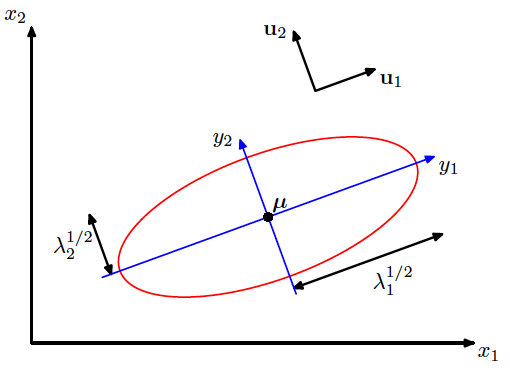再看在指数函数前面的部分，由$\Sigma =U\Lambda U'$可知$|\Sigma|=|\Lambda|$，因此$|\Sigma|^{-1}=|\Lambda|^{-1}=\prod\limits_{j=1}^{D}\lambda_j^{-1}$。
利用Jacobian matrix $J=U'$，并由$|J|^2=|U'U|=1$可得$|J|=1$，于是我们可以将原来的密度函数直接变换为
\begin{aligned} f_Y(y) =& |J|f_X\left(x(y)\right)\\ =& \dfrac{1}{(2\pi)^{D/2}}\dfrac{1}{\vert\Lambda\vert^{1/2}}\exp\left\{-\dfrac{1}{2}y'\Lambda^{-1}y\right\}\\ =& \prod_{j=1}^{D} \dfrac{1}{(2\pi \lambda_j)^{1/2}}\exp(-\dfrac{y_j^2}{2\lambda_j}) \end{aligned}
在原来的$x$坐标系下，各分量之间可能有相关性，对应二维时候的图像为下图（1），而在新的$y$坐标系下，协方差矩阵变为对角矩阵，也因此图像变为下图中（2）的情况，而若各分量同方差，则会变为类似于标准正态分布的同心圆即下图（3）的形式：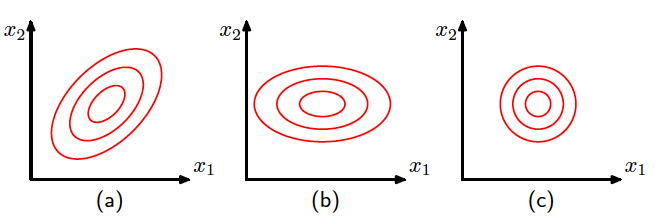展开全文统计学 统计模型 线性代数
• mvnfast ：快速多元正态分布和学生t分布 mvnfast R软件包提供了与多元正态分布和Student t分布有关的高效计算工具。 由于通过Rcpp \ RcppArmadillo软件包使用了C ++代码并通过OpenMP API进行了并行化，因此这些工具...R
• 标准正态分布的概率密度公式正态分布概率密度公式多元正态分布的概率密度公式上式为 x 服从 k 元正态分布，x 为 k 维向量；|Σ| 代表协方差矩阵的行列式。二维正态分布概率密度函数为钟形曲面，等高线是椭圆线族，...
标准正态分布的概率密度公式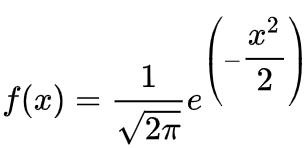正态分布概率密度公式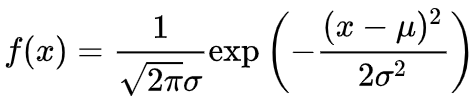多元正态分布的概率密度公式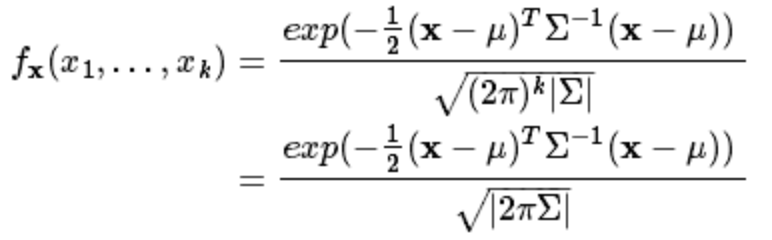上式为 x 服从 k 元正态分布，x 为 k 维向量；|Σ| 代表协方差矩阵的行列式。二维正态分布概率密度函数为钟形曲面，等高线是椭圆线族，并且二维正态分布的两个边缘分布都是一维正态分布，如图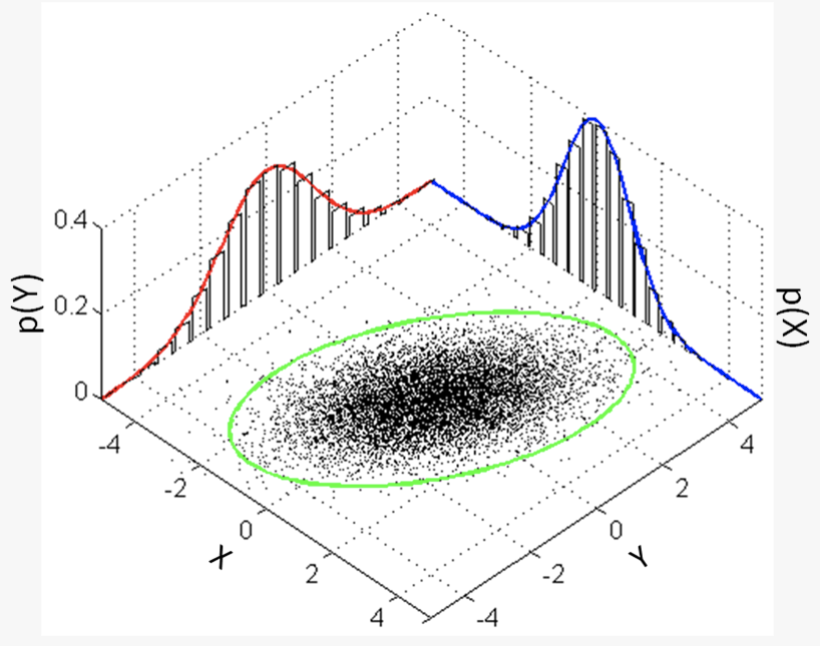numpy生成一个服从多元正态分布的数组multivariate_normal(mean, cov, size=None, check_valid=None, tol=None)各参数含义：mean：均值，维度为1，必选参数；cov：协方差矩阵，必选参数；size： 指定生成矩阵的维度，若size=(1, 1, 2)，则输出的矩阵的 shape 即形状为 1X1X2XN（N为mean的长度）；check_valid：可取值 warn，raise以及ignore；tol：检查协方差矩阵奇异值时的公差，float类型。示例：import numpy as npimport matplotlib.pyplot as pltmean = (1, 1)cov = np.array([[0.1, 0], [0, 1]])x = np.random.multivariate_normal(mean, cov, (500,), 'raise')   # nx2plt.scatter(x[:, 0], x[:, 1])plt.xlim(-3, 5)plt.ylim(-3, 5)plt.show()运行结果：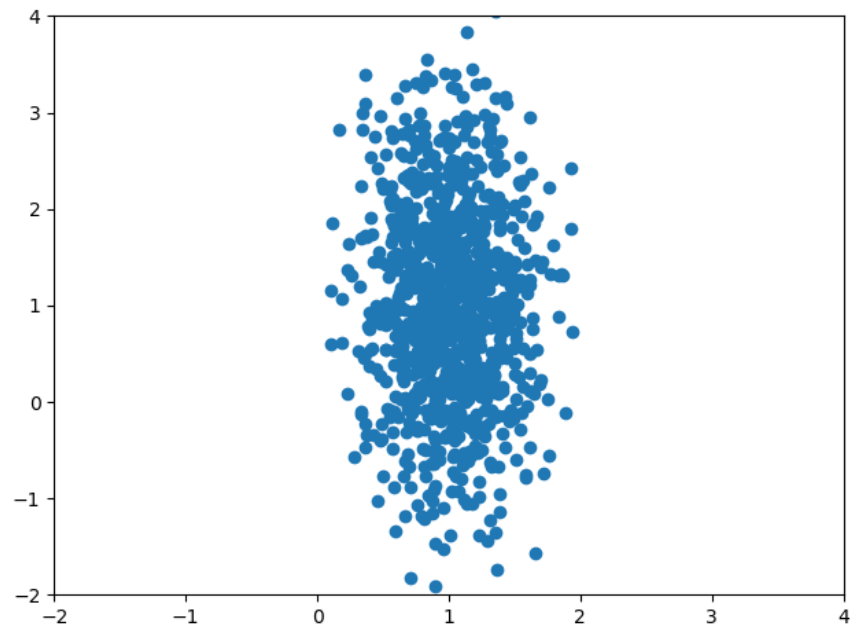参考资料
展开全文• 对于两个随机变量 XXX, YYY，若它们服从二维正态分布，则概率密度函数为： f(x,y)=12πσXσY1−ρ2exp⁡(−11−ρ2[(x−μX)2σX+(y−μY)2σY−2ρ(x−μX)(y−μY)σXσY]) f(x,y)=\frac{1}{2\pi \sigma_X\sigma_...
• 本文讨论了多元正态分布的定义，重点讨论多元正态分布的独立性、回归与最佳预测等问题。
• 算是一个学习指引吧，方便你找资料，其中也有部分底层代码，以及计算协方差的代码，代码都是经过验算的。涉及多元正态分布验算的部分，可以去寻找一元正态分布验算的代码，从而从曲线图像上观测样本拟合程度
• 文章目录3.1 多元正态分布的定义二元正态分布例子 3.1 多元正态分布的定义 N(μ,σ2)N(\mu,\sigma^2)N(μ,σ2) f(x)=12πσe−(x−μ)22σ2f(x)=\frac{1}{\sqrt{2\pi}\sigma}e^{-\frac{(x-\mu)^2}{2\sigma^2}}f(x)...
• 多元正态分布的概率密度函数 N维随机向量 如果服从多变量正态分布，必须满足下面的三个等价条件： 任何线性组合 服 从正态分布。 存在随机向量 ( 它的每个元素服从独立标准正态分布），向量 及 矩阵A满足 存在 和一...
• 原文来自师兄的博客：...多元正态分布多元正态分布的密度函数如下 ： fx(x1,...xn)=1(2π)k√|Σ|1/2exp(−12(x−μ)TΣ−1(x−μ))f_{x}(x_{1},...x_{n})=\frac{1}{\sqrt{(2\pi)^{条件概率密度
• 1 ，多元正态分布 ： 数据 代码 ： x, y = np.random.multivariate_normal([0, 0], [[1, -.5], [-.5, 1]], size=300).T 2 ，核密度估计图 ：sns.kdeplot 含义 ： 根据已有数据，画图连线，推测数据走向 3 ，...python
• 多元统计分析上机题之R语言实现（多元正态分布）-附件资源
• 多元正态分布、聚类分析（系统、模糊、k均值、有序样品）...